# Classic Machine Learning

### ASSOCIATION RULES

1. 1.
Association rules slides - apriori, eclat, fp growth - pretty complete
2. 2.
Terms - lift, confidence
Knoldus
1. 1.
Apriori
2. 3.
Fp-growth
APRIORI
1. 1.
2. 6.
1. 1.
Apriori
2. 2.
Rules
3. 3.
pgrowth
4. 4.
fpmax
FP Growth
1. 2.
The same example, but with a graph that shows that lower support cost less for fp-growth in terms of calc time.
2. 4.
Another clip video
3. 5.
How to validate these algorithms - probably the best way is confidence/support/lift
It depends on your task. But usually you want all three to be high.
• high support: should apply to a large amount of cases
• high confidence: should be correct often
• high lift: indicates it is not just a coincidence

### PROBABILISTIC ALGORITHMS

#### BAYES, BAYESIAN BELIEF NETWORKS

1. 2.
Introduction To BBS - a very good blog post
2. 3.
A complementing SLIDE presentation that shows how to build the network’s tables
3. 4.
A very nice presentation regarding BBS
4. 5.
5. 6.
Maximum Likelihood (log likelihood) - proofs for bernoulli, normal, poisson.

#### MARKOV MODELS

Random vs Stochastic (here and here):
• A variable is 'random'.
• A process is 'stochastic'.
Apart from this difference the two words are synonyms
In other words:
• A random vector is a generalization of a single random variables to many.
• A stochastic process is a sequence of random variables, or a sequence of random vectors (and then you have a vector-stochastic process).
(What is a Markov Model?) A Markov Model is a stochastic(random) model which models temporal or sequential data, i.e., data that are ordered.
• It provides a way to model the dependencies of current information (e.g. weather) with previous information.
• It is composed of states, transition scheme between states, and emission of outputs (discrete or continuous).
• Several goals can be accomplished by using Markov models:
• Learn statistics of sequential data.
• Do prediction or estimation.
• Recognize patterns.
(sunny cloudy explanation) Markov Chains is a probabilistic process, that relies on the current state to predict the next state.
• to be effective the current state has to be dependent on the previous state in some way
• if it looks cloudy outside, the next state we expect is rain.
• If the rain starts to subside into cloudiness, the next state will most likely be sunny.
• Not every process has the Markov Property, such as the Lottery, this weeks winning numbers have no dependence to the previous weeks winning numbers.
1. 1.
They show how to build an order 1 markov table of probabilities, predicting the next state given the current.
2. 2.
Then it shows the state diagram built from this table.
3. 3.
Then how to build a transition matrix from the 3 states, i.e., from the probabilities in the table
4. 4.
Then how to calculate the next state using the “current state vector” doing vec*matrix multiplications.
5. 5.
Then it talks about the setting always into the rain prediction, and the solution is using two last states in a bigger table of order 2. He is not really telling us why the probabilities don't change if we add more states, it stays the same as in order 1, just repeating.

#### MARKOV MODELS / HIDDEN MARKOV MODEL

HMM tutorials
1. 1.
HMM tutorial
1. 1.
Part 1, 2, 3, 4
2. 2.
Medium
1. 1.
Intro to HMM / MM
HMM variants
1. 2.
HMM LEARN (sklearn, still being developed)
2. 3.
Pomegranate (this is good)
1. 1.
General mixture models
2. 2.
Hmm
3. 3.
Basyes classifiers and naive bayes
4. 4.
Markov changes
5. 5.
Bayesian networks
6. 6.
Markov networks
7. 7.
Factor graphs
3. 5.
Hmms (old)
HMM (what is? And why HIDDEN?) - the idea is that there are things that you CAN OBSERVE and there are things that you CAN'T OBSERVE. From the things you OBSERVE you want to INFER the things you CAN'T OBSERVE (HIDDEN). I.e., you play against someone else in a game, you don't see their choice of action, but you see the result.
1. 1.
Python code, previously part of sklearn
2. 2.
Python seqLearn - supervised multinomial HMM
This youtube video part1 - explains about the hidden markov model. It shows the visual representation of the model and how we go from that the formula: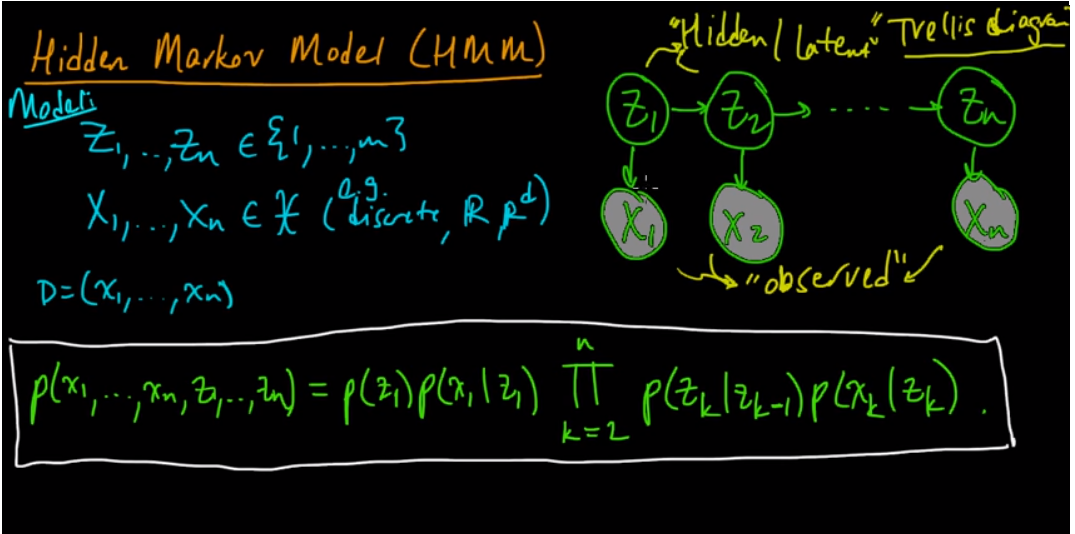It breaks down the formula to:
• transition probability formula - the probability of going from Zk to Zk+1
• emission probability formula - the probability of going from Zk to Xk
• (Pi) Initial distribution - the probability of Z1=i for i=1..m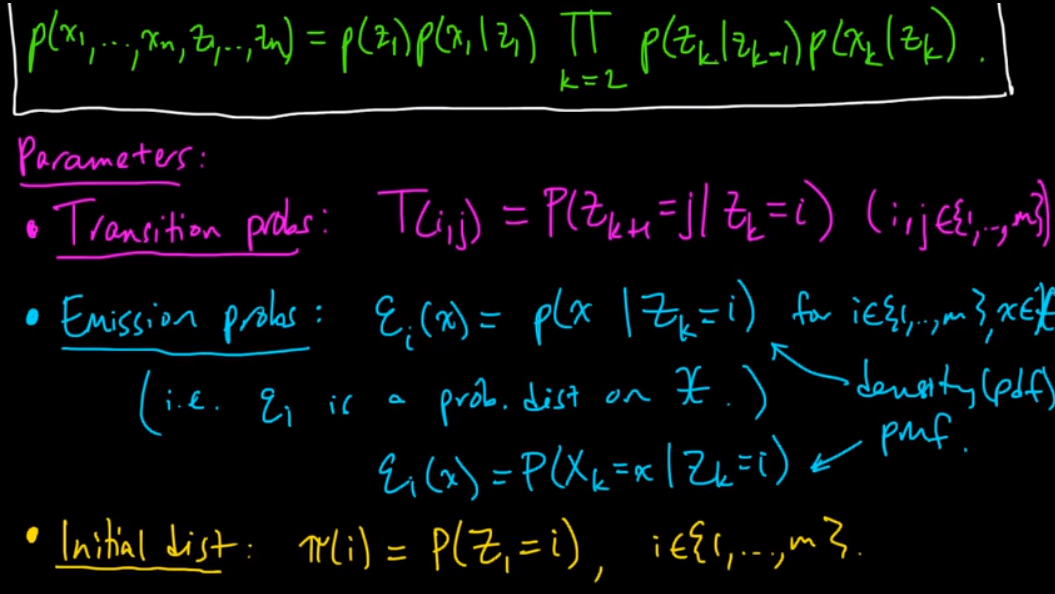In part2 of the video:
1. 1.
Probably the simplest explanation of Markov Models and HMM as a “game” - link
2. 2.
This video explains that building blocks of the needed knowledge in HMM, starting probabilities P0, transitions and emissions (state probabilities)
3. 3.
This post, explains HMM and ties our understanding.This is the iconic image of a Hidden Markov Model. There is some state (x) that changes with time (markov). And you want to estimate or track it. Unfortunately, you cannot directly observe this state (hidden). That's the hidden part. But, you can observe something correlated with the state (y).
OBSERVED DATA -> INFER -> what you CANT OBSERVE (HIDDEN).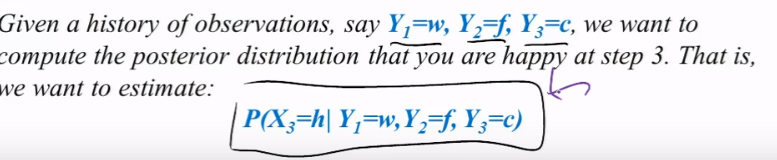Considering this model:
• where P(X0) is the initial state for happy or sad
• Where P(Xt | X t-1) is the transition model from time-1 to time
• Where P(Yt | Xt) is the observation model for happy and sad (X) in 4 situations (w, sad, crying, facebook)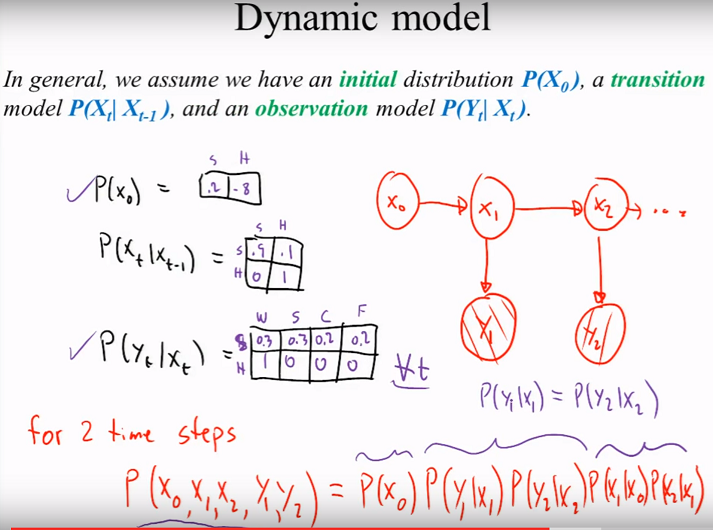#### INPUT OUTPUT HMM (IOHMM)

1. 1.
Incomplete python code for unsupervised / semi-supervised / supervised IOHMM - training is there, prediction is missing.

#### CONDITIONAL RANDOM FIELDS (CRF)

1. 4.
Neural network CRF NNCRF
2. 5.
3. 8.
Python crfsuite wrapper

### REGRESSION ALGORITHMS

1. 1.
Sk-lego to fit with intervals a linear regressor on top of non linear data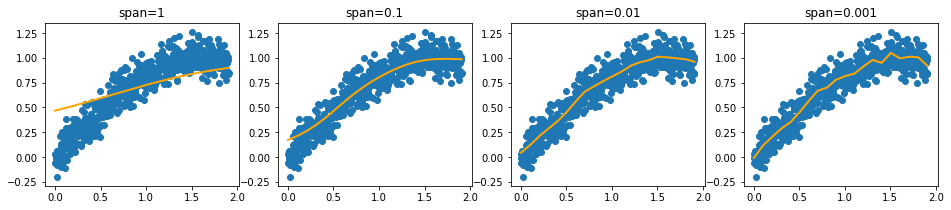1. 1.
Sk-lego monotonic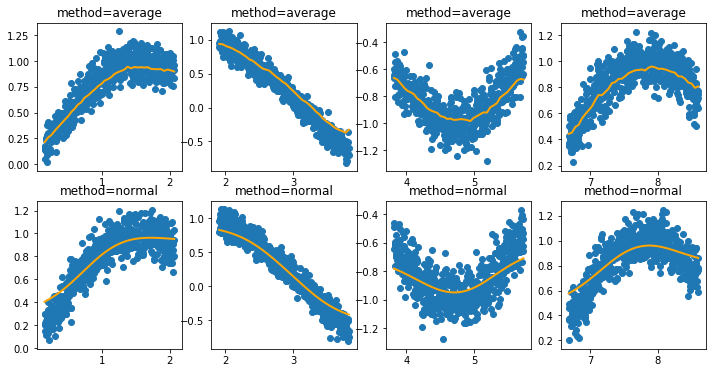1. 1.
Lightning - lightning is a library for large-scale linear classification, regression and ranking in Python.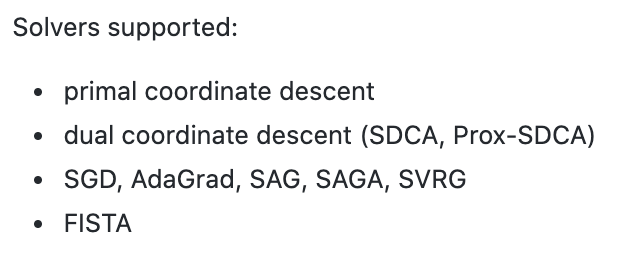2. 2.
Linear regression TBC
3. 3.
CART - classification and regression tree, basically the diff between classification and regression trees - instead of IG we use sum squared error
4. 4.
SVR - regression based svm, with kernel only.
5. 5.
NNR- regression based NN, one output node
6. 6.
LOGREG - Logistic regression - is used as a classification algo to describe data and to explain the relationship between one dependent binary variable and one or more nominal, ordinal, interval or ratio-level independent variables. Output is BINARY. I.e., If the likelihood of killing the bug is > 0.5 it is assumed dead, if it is < 0.5 it is assumed alive.
• Assumes binary outcome
• Assumes no outliers
• Assumes no intercorrelations among predictors (inputs?)
Regression Measurements:
1. 1.
1. 1.
Too many variables
2. 2.
Overfitting
3. 3.
Time series - seasonality trends can cause this
2. 2.

#### KERNEL REGRESSION

Gaussian Kernel Regression does–it takes a weighted average of the surrounding points
• variance, sigma^2. Informally, this parameter will control the smoothness of your approximated function.
• Smaller values of sigma will cause the function to overfit the data points, while larger values will cause it to underfit
• There is a proposed method to find sigma in the post!
• Gaussian Kernel Regression is equivalent to creating an RBF Network with the following properties: - described in the post

### ​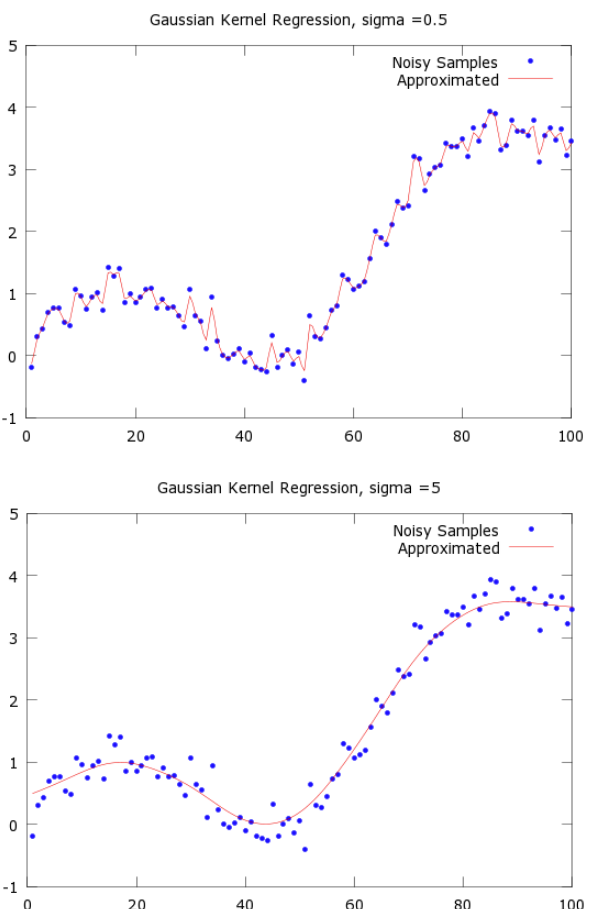​

#### DIMENSIONALITY REDUCTION

PRINCIPAL COMPONENT REGRESSION (PCR) / PARTIAL LEAST SQUARES (PLS)
Principal component regression (PCR) Partial least squares and (PLS) - basically PCA and linear regression , however PLS makes use of the response variable in order to identify the new features.
One can describe Principal Components Regression as an approach for deriving a low-dimensional set of features from a large set of variables. The first principal component direction of the data is along which the observations vary the most. In other words, the first PC is a line that fits as close as possible to the data. One can fit p distinct principal components. The second PC is a linear combination of the variables that is uncorrelated with the first PC, and has the largest variance subject to this constraint. The idea is that the principal components capture the most variance in the data using linear combinations of the data in subsequently orthogonal directions. In this way, we can also combine the effects of correlated variables to get more information out of the available data, whereas in regular least squares we would have to discard one of the correlated variables.
The PCR method that we described above involves identifying linear combinations of X that best represent the predictors. These combinations (directions) are identified in an unsupervised way, since the response Y is not used to help determine the principal component directions. That is, the response Y does not supervise the identification of the principal components, thus there is no guarantee that the directions that best explain the predictors also are the best for predicting the response (even though that is often assumed). Partial least squares (PLS) are a supervised alternative to PCR. Like PCR, PLS is a dimension reduction method, which first identifies a new smaller set of features that are linear combinations of the original features, then fits a linear model via least squares to the new M features. Yet, unlike PCR, PLS makes use of the response variable in order to identify the new features.